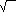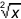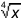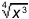Classrooms Curricula References Textbooks Site Map

# Fractional Exponents

Fractional exponents are just a mathematical shorthand for the "root symbol",. The number of the root becomes the denominator of the fractional exponent so= x1/2= x1/3= x1/4
etc.

A regular exponent goes into the numerator so= x3/4

Why would we want to replace the perfectly good root symbol with this different notation? Because, this notation lets us perform arithmetic with "roots" that we couldn't have done before. For example our rules for multiplying exponents tells us that

x1/2 · x1/2 = x1/2 + 1/4 = x3/4

Our root notation, on the other hand, gave us no way to go from·to.